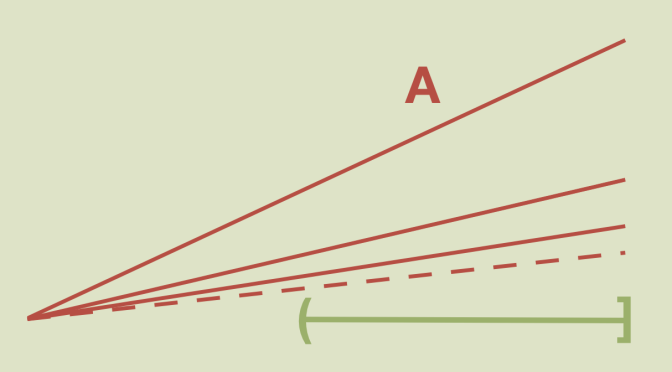# A connected not locally connected space

In this article, I will describe a subset of the plane that is a connected space while not locally connected nor path connected.

Let’s consider the plane $$\mathbb{R}^2$$ and the two subspaces:
$A = \bigcup_{n \ge 1} [(0,0),(1,\frac{1}{n})] \text{ and } B = A \cup (\frac{1}{2},1]$ Where a segment noted $$|a,b|$$ stands for the plane segment $$|(a,0),(b,0)|$$.

Let’s consider $$B$$ and $$\overline{B}$$ topological properties equipped with the subspace topology.

## $$A$$ and $$B$$ are connected

It is well know that a path connected space is connected. Hence $$A$$ is connected. If $$B$$ wasn’t connected, $$B$$ would be the disjoint union of two non empty open sets $$O_1$$ and $$O_2$$. As $$A$$ is connected, $$A$$ is the subset of $$O_1$$ or $$O_2$$. Let say $$A \subset O_1$$. Also, $$(\frac{1}{2},1]$$ is connected. Hence $$(\frac{1}{2},1] \subset O_2$$ as $$O_2 \neq \emptyset$$. Now any neighborhood of a point of $$(\frac{1}{2},1]$$ intersects $$A$$ which is a contradiction.

## We have $$\overline{B}=A \cup [0,1]$$ and $$\overline{B}$$ is connected

A point $$(x,0) \in [0,1]$$ is the limit of the sequence $$((x,\frac{x}{n}))_{n \ge 1}$$. Therefore $$\overline{B}=A \cup [0,1]$$. And $$\overline{B}$$ is connected as the closure of a connected set.

## $$\overline{B}$$ is path connected while $$B$$ is not

$$\overline{B}$$ is path connected as any point in $$\overline{B}$$ can be joined to the plane origin: consider the line segment joining the two points.
For the proof that $$B$$ is not path connected, suppose that $$\gamma$$ is a path joining the point $$(\frac{3}{4},0)$$ to the point $$(1,1)$$. We note $$\arg \gamma(t)$$ the angle of $$\overrightarrow{\gamma(t)}$$ with the X-axis. $$\arg \gamma(t)$$ is a continuous function of $$t \in [0,1]$$. Hence its range is connected (the image of a connected set under a continuous map is connected). This is in contradiction with the fact that $$\arg (1,1)$$ is an isolated point of $$\arg(B)$$.

## Neither $$B$$ nor $$\overline{B}$$ is locally connected

The point $$P=((\frac{3}{4},0)$$ belongs to $$B$$. The intersection $$U$$ of the open disk centered on $$P$$ with radius $$\frac{1}{4}$$ and $$B$$ is an open of $$B$$. As the origin doesn’t belong to $$U$$, $$B$$ and $$\overline{B}$$ are not locally connected at $$P$$.

Finally, $$B$$ is connected, not locally connected and not path connected.